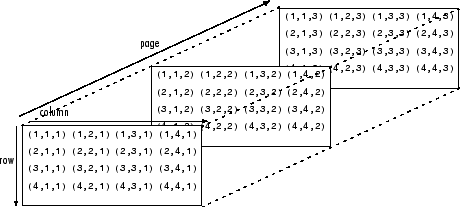# pagetranspose

Page-wise transpose

## Syntax

``Y = pagetranspose(X)``

## Description

example

````Y = pagetranspose(X)` applies the nonconjugate transpose to each page of N-D array `X`. Each page of the output `Y(:,:,i)` is found by transposing the corresponding page in `X`, as in `X(:,:,i).'`.```

## Examples

collapse all

Create a 3-D array `A`, and then use `pagetranspose` to transpose each page of the array.

```r = repelem(1:3,3,1); A = cat(3,r,2*r,3*r)```
```A = A(:,:,1) = 1 2 3 1 2 3 1 2 3 A(:,:,2) = 2 4 6 2 4 6 2 4 6 A(:,:,3) = 3 6 9 3 6 9 3 6 9 ```
`B = pagetranspose(A)`
```B = B(:,:,1) = 1 1 1 2 2 2 3 3 3 B(:,:,2) = 2 2 2 4 4 4 6 6 6 B(:,:,3) = 3 3 3 6 6 6 9 9 9 ```

## Input Arguments

collapse all

Input array, specified as a multidimensional array.

Data Types: `single` | `double` | `int8` | `int16` | `int32` | `int64` | `uint8` | `uint16` | `uint32` | `uint64` | `logical` | `char` | `string` | `struct` | `cell` | `categorical` | `datetime` | `duration` | `calendarDuration`
Complex Number Support: Yes

collapse all

### Array Pages

Page-wise functions like `pagetranspose` operate on 2-D matrices that have been arranged into a multidimensional array. For example, with a 3-D array the elements in the third dimension of the array are commonly called pages because they stack on top of each other like pages in a book. Each page is a matrix that gets operated on by the function.You can also assemble a collection of 2-D matrices into a higher dimensional array, like a 4-D or 5-D array, and in these cases `pagetranspose` still treats the fundamental unit of the array as a 2-D matrix that gets operated on, such as `X(:,:,i,j,k,l)`.

The `cat` function is useful to assemble a collection of matrices into a multidimensional array, and the `zeros` function is useful to preallocate a multidimensional array.

## Tips

• The page-wise transpose is equivalent to permuting the first two dimensions of the array with `permute(X,[2 1 3:ndims(X)])`.

## Version History

Introduced in R2020b